# Conditional Generative Model

Conditional Generative Model

## Supervised Conditional

• Use paired data to train the network

### Conditional GAN

• Reason to add noise: Without noise, the network only learn the map from the input (e.g. text ‘train’, ‘car’, etc.) to the average of multiple positive samples (e.g. the average of the front and side train), which is very bad.

• Generator: take a normal (Gaussian) distribution and a constraint as inputs
• Discriminator: take generated output and a constraint as inputs, to check both:
• if the result is realistic
• if the result matches the constraint
• Algorithm:
1. Sample m examples $\{ (c^1, x^{1}), (c^2, x^{2}), \ldots, (c^m, x^{m}) \}$ from database
2. Sample m noise samples $\{z^{1}, z^{2}, \ldots, z^{m}\}$ from a distribution
3. Obtaining generated data $\{\tilde{x}^{1}, \tilde{x}^{2}, \ldots, \tilde{x}^{m}\}, \tilde{x}^{i}=G\left(z^{i}\right)$
4. Sample m objects $\{\hat{x}^{1}, \hat{x}^{2}, \ldots, \hat{x}^{m}\}$ from database
5. Update discriminator paramters $\theta_{d}$ to maximize
\begin{aligned} &\tilde{V}=\frac{1}{m} \sum_{i=1}^{m} \log D\left(c^{i}, x^{i}\right)+\frac{1}{m} \sum_{i=1}^{m} \log \left(1-D\left(c^{i}, \tilde{x}^{i}\right)\right)+\frac{1}{m} \sum_{i=1}^{m} \log \left(1-D\left(c^{i}, \hat{x}^{i}\right)\right) \\ &\theta_{d} \leftarrow \theta_{d}+\eta \nabla \tilde{V}\left(\theta_{d}\right) \end{aligned}
6. Sample m noise samples $\{z^{1}, z^{2}, \ldots, z^{m}\}$ from a distribution
7. Sample m conditions $\{c^{1}, c^{2}, \ldots, c^{m}\}$ from a database
8. Update generator parameters $\theta_{g}$ to maximize
$\tilde{V}=\frac{1}{m} \sum_{i=1}^{m} \log \left(D\left(G\left(c^{i}, z^{i}\right)\right)\right), \theta_{g} \leftarrow \theta_{g}-\eta \nabla \tilde{V}\left(\theta_{g}\right)$

#### Discriminator

There are two commonly used prototype of structure of Discriminator:

1. Use two different network to receive object and condition. Green Network receive the object encoding and condition embedding and output final result which represent if generation is realistic and matched or not.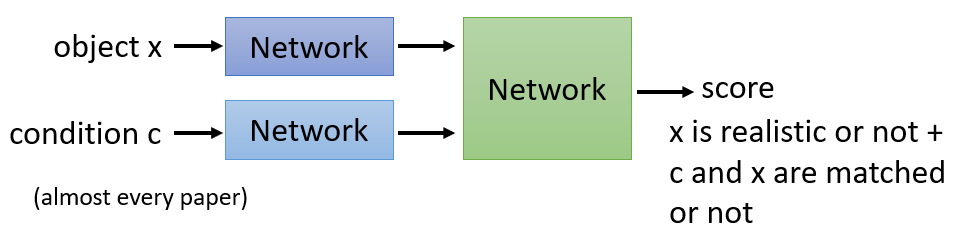2. Two network output different scores. Blue network receives object encoding and condition to output match result.• The first structure may confuse between the bad generation and bad matching.

#### Stack GAN

Progressively generate large resolution image by stacking generator.

• low resolution generator take embedding and noise to output small image
• The small images from the previous generator will be taken as input, replacing the noise, to refine the detail and enlarge the resolution.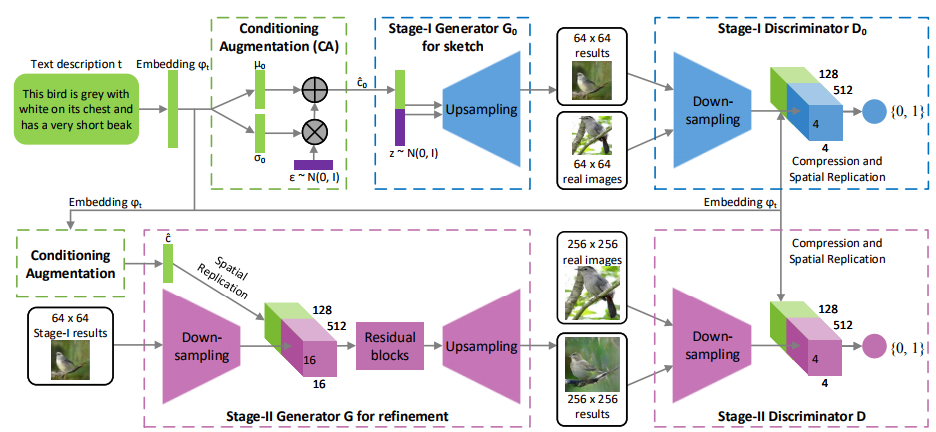*reference-3

#### Image-to-image

1. Non-adversarial approach: (Traditional supervised approach using autoencoder) directly mapping from the input to image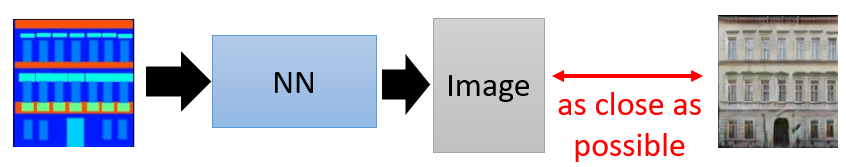The result is blurry because as stated in Comparing to VAE, it is the average of several samples.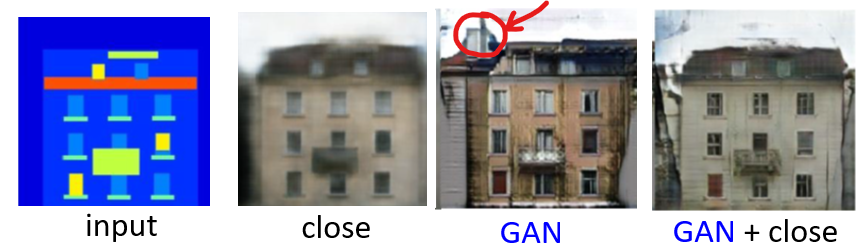2. Use GAN to generate: generated image and conditions are fed into discriminator to judge realisitc or not. At the same time, close constraint is to guide generator to output as closed to the paired image in the training sample as possible.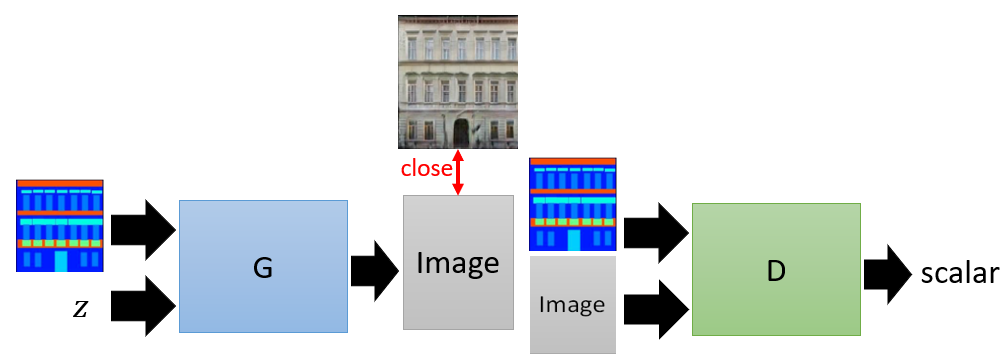• As the above figure shows, without close constraint part, which is implemented in L1 distance to measure the difference between results and paired real images, the output will be more diversed but something unrelated to the original input will be generated.

TODO

### Speech Enhancement

• More generally, the paired samples can be adopted to train GAN, e.g. noisy speech and clean voice pair.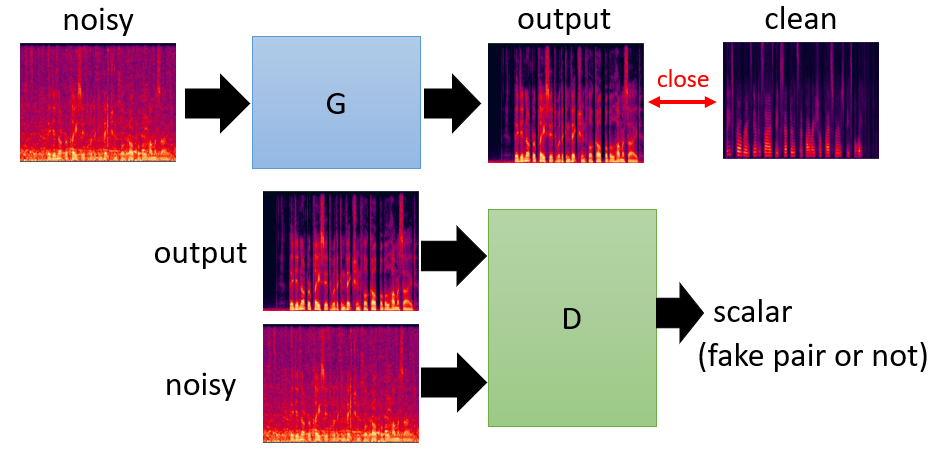### Video Generation

• Generator predict the future frame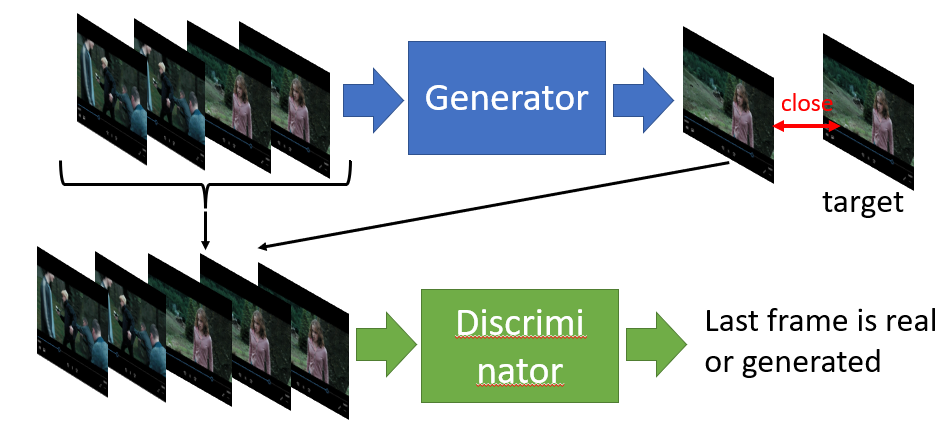## Unsupervised Conditional

• Paired data for training is expensive or difficult to build.
• Different drawing style with the same contents.
• Same sentence spoke by male and female.
• Unsupervised conditional generation: transform from one domain to another without paired data
• Approach 1: direct transformation
• change the texture or color, low level modification using shallow generator
• Approach 2: Projection to a common space
• only keep semantics

### Direction Transformation

• Transform the input image from domain $X$ directly to domain $Y$.
• Discriminator distinguishes if the generated image belongs to domain $Y$.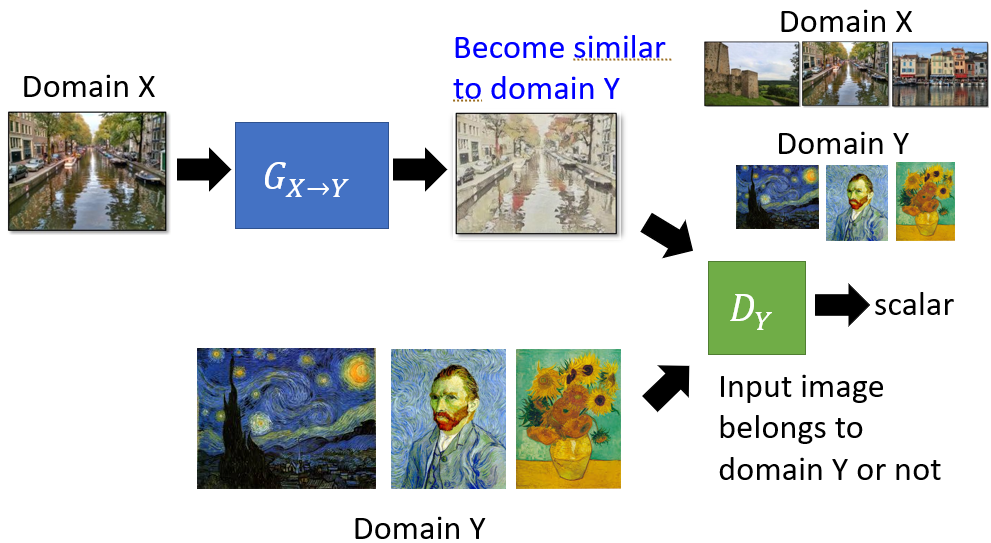• This approach may lead to the generated image closed to domain $Y$ but not similar to the original image, since discriminator still believes results belong to $Y$ in this case.
• This can be avoided by generator design: simpler generator makes the input and output more closely related.

*reference-4

• One workaround: use other module to guide the network output the image with the similar semantic representation
• Backbone is feature extractor, such as VGG, which is pretrained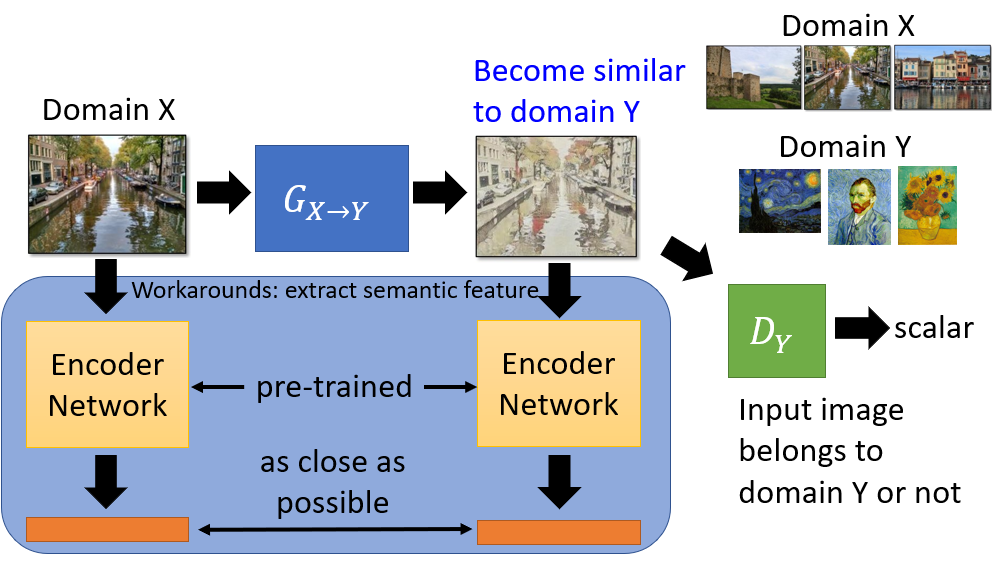*reference-5

#### CycleGAN

• CycleGAN is another solution, based on cycle consistency loss transforming the images between two domains.
\begin{aligned} \mathcal{L}_{\mathrm{GAN}}\left(G_X, D_{Y}, X, Y\right) &=\mathbb{E}_{y \sim p_{\text {data }}(y)}\left[\log D_{Y}(y)\right]+\mathbb{E}_{x \sim p_{\text {data }}(x)}\left[\log \left(1-D_{Y}(G_X(x))\right]\right. \\ \mathcal{L}_{\mathrm{GAN}}\left(G_Y, D_{X}, Y, X\right) &=\mathbb{E}_{x \sim p_{\text {data }}(x)}\left[\log D_{X}(x)\right]+\mathbb{E}_{y \sim p_{\text {data }}(y)}\left[\log \left(1-D_{X}(G_Y(y))\right]\right. \\ \mathcal{L}_{\text {cyc }}(G_X, G_Y) &=\mathbb{E}_{x \sim p_{\text {data }}(x)}\left[\|G_Y(G_X(x))-x\|_{1}\right]+\mathbb{E}_{y \sim p_{\text {data }}(y)}\left[\|G_X(G_Y(y))-y\|_{1}\right] \\ \mathcal{L}\left(G_X, G_Y, D_{X}, D_{Y}\right) &=\mathcal{L}_{\mathrm{GAN}}\left(G_X, D_{Y}, X, Y\right)+\mathcal{L}_{\mathrm{GAN}}\left(G_Y, D_{X}, Y, X\right) +\lambda \mathcal{L}_{\mathrm{cyc}}(G_X, G_Y) \\ G_X^{*}, G_Y^{*} &= \arg \min _{G_X, G_Y} \max _{D_{X}, D_{Y}} \mathcal{L}\left(G_X, G_Y, D_{X}, D_{Y}\right) \end{aligned}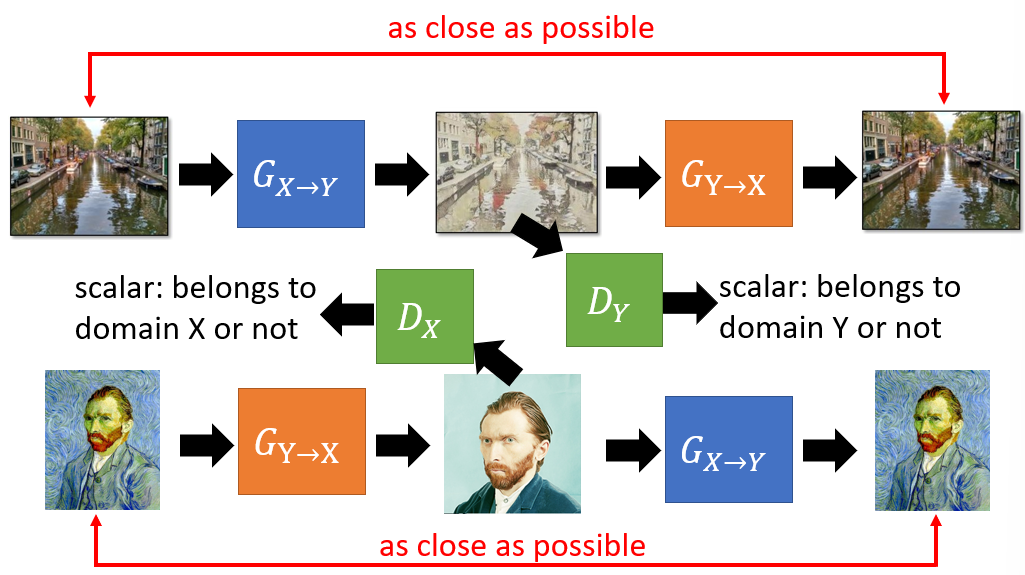*reference-6

• The problem of CycleGAN: a master of steganography (信息隐藏), which means some information is hidden through the first generator in a way that is invisible to human eyes (e.g. black spot becomes inconspicuous) but restored after the second generator.
• It is easier for the generator to get a high score from the discriminator by hiding some information.
• In this scene, cycle consistency is not promised because domain transformation is achieved by retaining some invisible details in the output image from the first generator.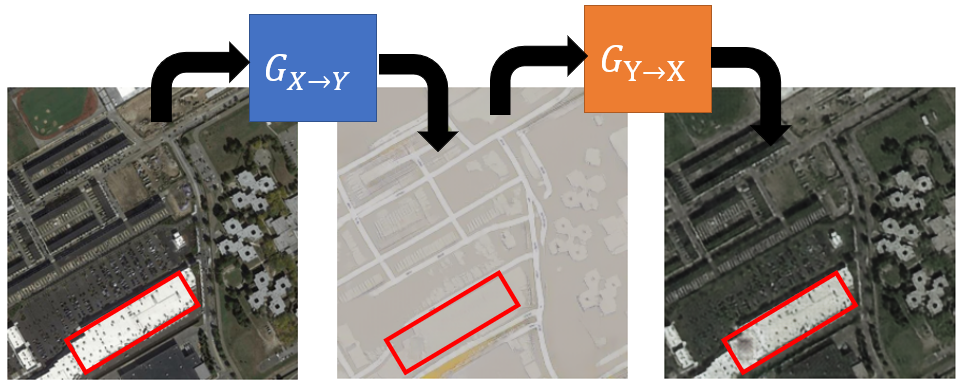*reference-7

TODO

TODO

#### StarGAN

• For previous work, multiple models should be built independently for each pair of domains.
• StarGAN extends the scalability of cycleGAN in handling more than two domains by using only one generator.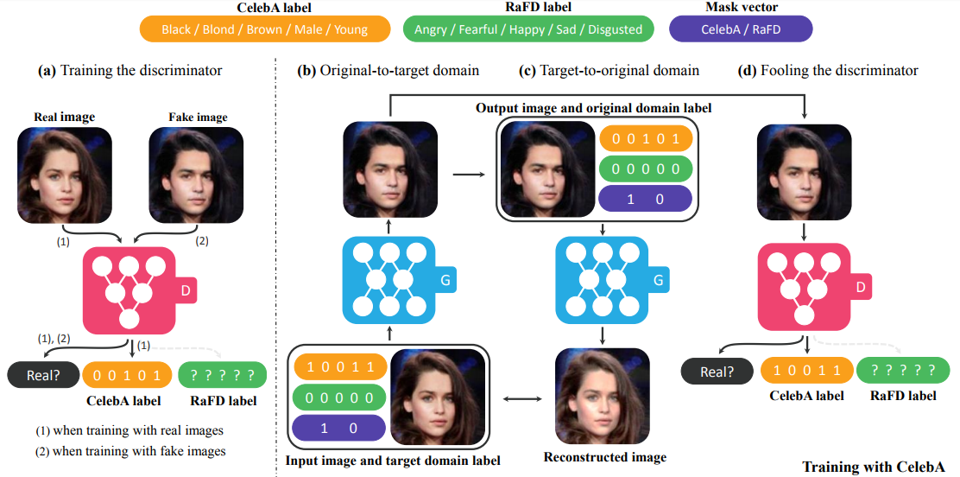*reference-9

### Projection to a common space

• Instead of mapping the image of domain $X$ directly to domain $Y$, this firstly encode the image into the latent space which will be then projected to another domain by decoder.

#### Common problem and workarounds

• Reconstruction error is built to guide each branch of network can reconstruct images in each domain after the encoding.

• Discriminator optimize the generation quality and solve obscure images produced by autoencoder (learn by averaging)

• After separately training, each row of the network has ability to reconstruct image in each domain.

• But images with the same attribute in different domain may not project to the same position in the latent space.
• The distribution of the latent representations from different domain are different.
• For example, black hair in the domain $A$ may be projected to [0, 0, 1] by $EN_X$, but black hair in the domain $B$ may be projected to [1, 0, 0] by $EN_Y$.
• We can not get output with desirable features by controlling the latent space vector in inference stage.
• It doesn’t make sense to train the latent space in this way!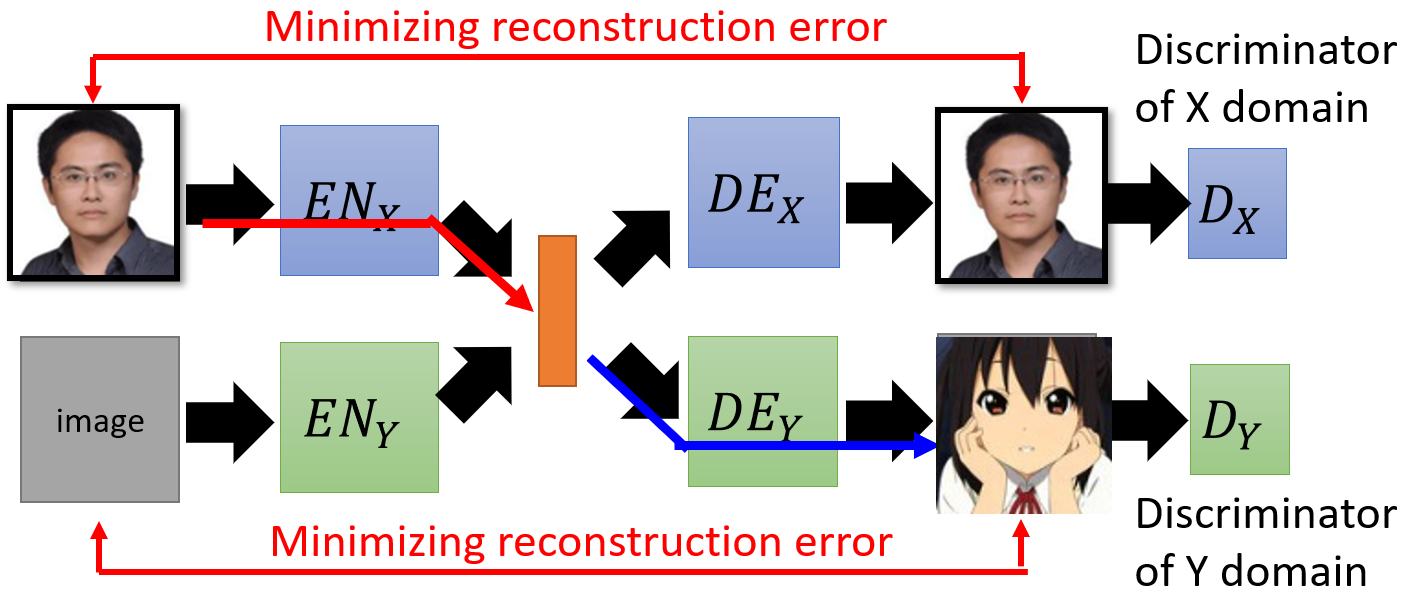#### Couple GAN && UNIT

TODO Couple GAN, UNIT

• Couple GAN and UNIT share the parameters of last several layers of encoders and firsst several layers of decoders, which ensures that different encoders/decoders has exact same mapping function to the latent space.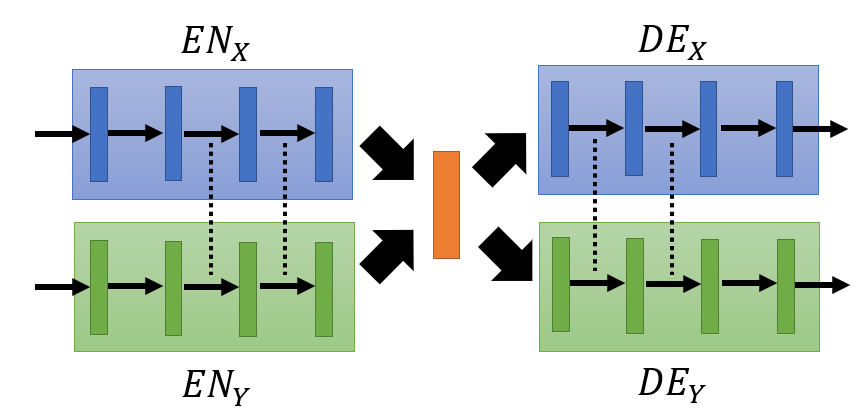*reference-10, *reference-11

• Comparing to sharing the parameters of the network, Domain Discriminator is built to trained adversarially to disentange the latent representation directly.
• $EN_X$ and $EN_Y$ are trained to fool the domain discriminator.
• Domain Discriminator distinguishes the latent code from $EN_X$ or $EN_Y$, forcing the output of $EN_X$ and $EN_Y$ have the same distribution.
• Well-trained Fader Network: $EN_X$ and $EN_Y$ map the images from different domain to the same distribution as latent representations.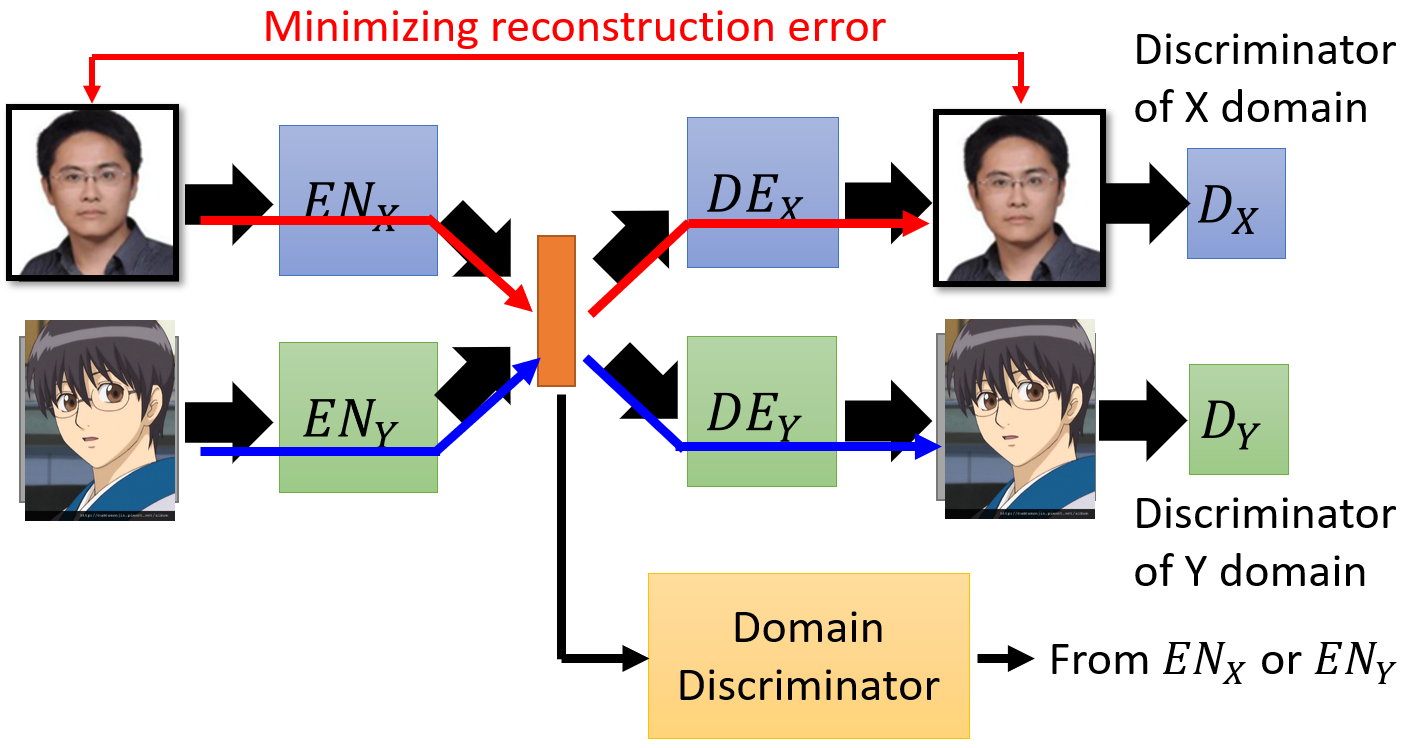*reference-12

#### ComboGAN

• In the same way that CycleGAN uses unsupervised learning and cycle consistency loss, ComboGAN adds latent vectors to control generation features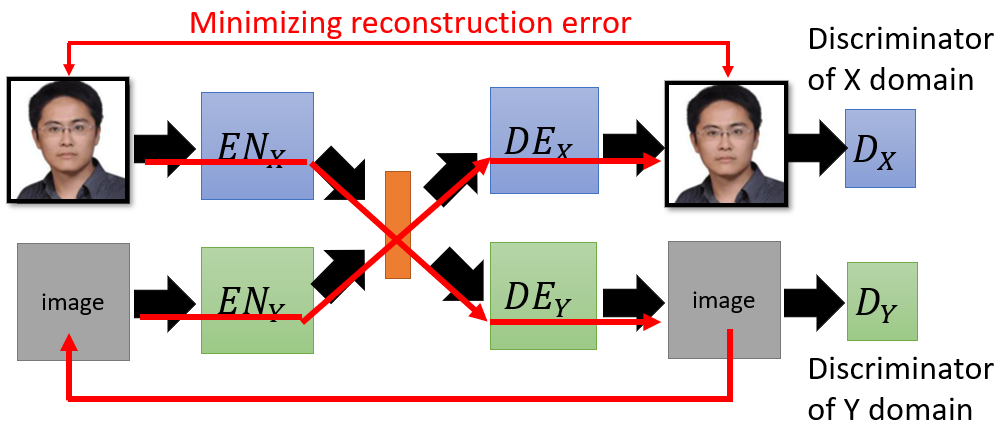*reference-13

TODO ComboGAN

#### XGAN && DTN

• Comparing to ComboGAN do cycle-consistency loss in pixel wise, XGAN and DTN guide the encoders mapping to the same latent space by the semantic-consistency loss on the latent representation itself.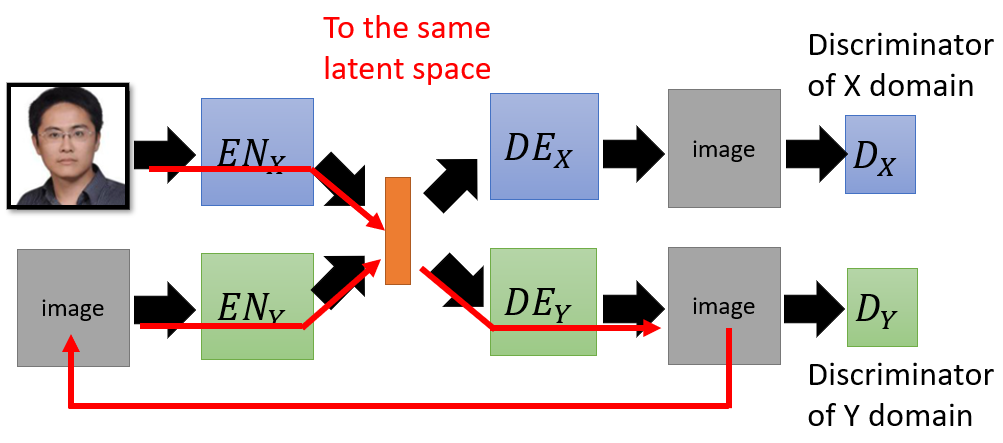*reference-14

TODO XGAN TODO DTN: unsupervised

TODO Bram matrix